# Interpreting Graphs and Charts of Scientific Data: Practice Problems

Lesson Transcript
Instructor: April Koch

April teaches high school science and holds a master's degree in education.

To interpret charts and graphs of scientific data, you should know the correct orientation of x- and y-axes, understand the trends of the chart, and answer the chart questions on your own. Learn more about the three rules of solving problems that include charts and graphs, and explore relevant practice problems. Updated: 08/17/2021

## Solving Problems with Charts and Graphs

Have you ever been in the middle of a test, happily going along question-by-question, trying to pace yourself, and all of a sudden - wham - they smack you with a huge, scary graph and a whole page of questions about it? It takes you five minutes just to read the questions through and another five minutes to try and interpret the graph. Complex chart problems are just as stressful as table problems during an exam. But, just like you learned in our lesson on table problems, there are certain tactics that can help you get by. In this lesson, we'll walk through two chart problems and learn three simple rules for solving them efficiently.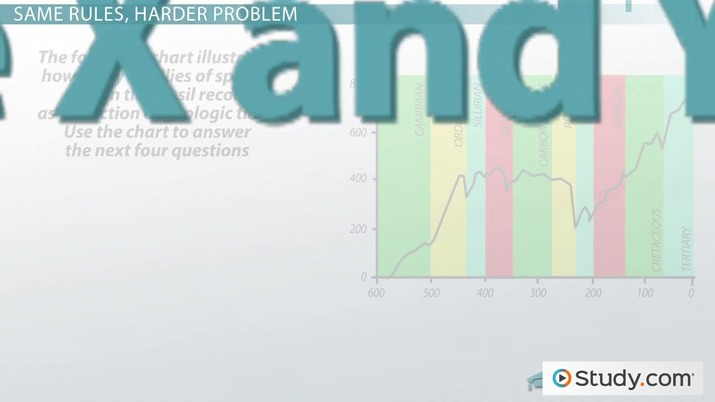An error occurred trying to load this video.

Try refreshing the page, or contact customer support.

Coming up next: Frederick Griffith: Experiment & Discovery

### You're on a roll. Keep up the good work!

Replay
Your next lesson will play in 10 seconds
• 0:41 Three Rules for Chart Problems
• 4:16 Applying the Rules to…
• 8:29 Lesson Summary
Save Save

Want to watch this again later?

Timeline
Autoplay
Autoplay
Speed Speed

## Three Rules for Chart Problems

Our first chart problem describes a situation with enzymes. Let's see what it says. 'The graph below illustrates the percentage of maximum activity for three different enzymes over a range of pH levels. Use the graph to answer the following three questions.'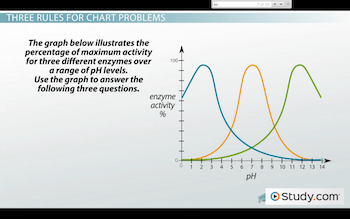The first thing to do with a problem like this is to orient yourself to the x- and y-axes. Above is the line graph that the problem provides. Before you read through any of the questions, it's a good idea to read the axes titles and make sure you understand how the graph is supposed to work. The x-axis here is titled 'pH.' We can see that the graph covers the full range of pH levels, from 0 to 14. The y-axis measures enzyme activity in terms of percentages. When one of the lines reaches the height of the graph, that means that the enzyme represented by that line reaches the highest activity level at that pH.

Now that we've oriented ourselves to the axes, we need to identify the trends of the chart. We need to be sure we understand the relationships that are represented by the lines in the graph. This will usually require us to remember the relevant material from our previous science studies. We already know that different enzymes peak in their activity at different pHs. This graph is just showing that relationship for three hypothetical enzymes. The first enzyme, A, reaches its highest activity at a low pH, like 3 or 4. Enzyme B peaks at a pH of 7, and enzyme C looks like it peaks around pH 11 or 12. The three lines cross over one another at intermediate pH levels. So at the pHs of 5 and 9, none of the enzymes are all that active.

Alright, so we have a good understanding of what this graph is about. Now, we can go ahead and look at the questions. Our first question asks, 'Which enzyme does best at pH 3?' So, looking at our graph, we'll find pH 3 along the x-axis. Move straight up to follow the y-axis, and we intersect with the line that corresponds to enzyme A. That was easy! We'll pick enzyme A.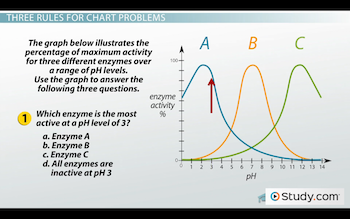The next question asks us to do the same thing, only backwards. Instead of finding the enzyme that peaks at a certain pH, we need to find the pH at which a certain enzyme peaks. Our question reads, 'What is the optimum pH for enzyme C?' You know that the optimum pH for an enzyme is the pH level at which it reaches maximum activity. So let's find the graph for enzyme C, locate the highest point, and then move vertically downward to see where we end up on the x-axis. We end up right around pH 11. So, we'll choose pH 11 from our list of choices.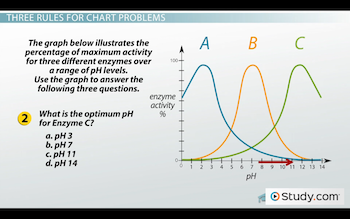Did you notice that in both questions, we found our own answer before looking at any of the choices? It's usually best to answer the question on your own when you're solving complex chart problems. If you come up with the right answer before you look at the other choices, you'll save yourself from becoming distracted, or even swayed, by the other options available. Let's try this tactic with our third question. It reads, 'What is the order of enzyme activity, from greatest to least, at a pH of 8?' Let's look at what's going on at pH 8 in our graph. If we go up from the x-axis, we first cross the line for enzyme A, then C, then B. So that means at a pH level of 8, enzyme B is the most active, enzyme C is less active and enzyme A is the least active. Our answer to this question, without looking at any of the choices, would be enzyme B, then C, then A. Now, is that answer listed as one of our choices? Yup, it's right here. So, we circle that answer and now we've done the problem!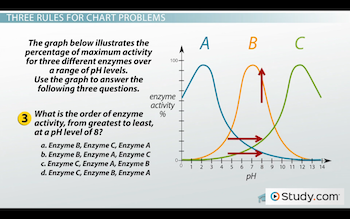## Same Rules, Harder Problem

Next, we'll try a chart problem that's a little bit harder. Here's what it says: 'The following chart illustrates how many families of species exist in the fossil record as a function of geologic time. Use the chart to answer the next four questions.'

This problem may look like it's going to take a lot of work. But, remember that before you read through any of the questions, you should first orient yourself to the x- and y-axes. The x-axis here reads 'Geologic Time in Millions of Years Ago.' That word 'ago' should tell you something important. Notice that the numbers down here run backwards, with the zero at the right-hand side of the graph. Instead of counting up the years from left to right, we're counting back the years from right to left. This chart sort of functions as a timeline. And look, down here we have the different periods of geologic history labeled and sectioned off for us. The y-axis here reads 'Number of Families.' They must be talking about families in a phylogenic sense. Rather than tracking the number of species that existed through time, they've tracked organisms in larger groups - families.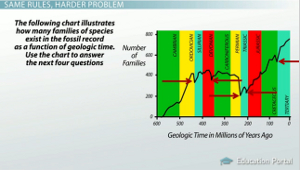To unlock this lesson you must be a Study.com Member.

### Register to view this lesson

Are you a student or a teacher?